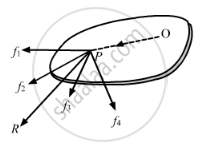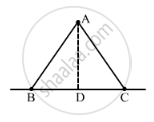Advertisement Remove all ads

# Three Particles, Each of Mass 200 G, Are Kept at the Corners of an Equilateral Triangle of Side 10 Cm. Find the Moment of Inertial of the System About an Axis Joining Two of the Particles. - Physics

Sum

Three particles, each of mass 200 g, are kept at the corners of an equilateral triangle of side 10 cm. Find the moment of inertial of the system about an axis joining two of the particles.Advertisement Remove all ads

#### Solution

The distance of mass at A from the axis passing through side BC,

$\left( AD \right) = \frac{\sqrt{3}}{2} \times 10 = 5\sqrt{3} cm$Therefore, we have

Moment of inertia of mass about the axis BC,

$l = m r^2 = 200 \times \left( 5\sqrt{3} \right)^2$

$= 200 \times 25 \times 3$

$= 15000 gm - {cm}^2$

$= 1 . 5 \times {10}^{- 3} kg - m^2$

Concept: Values of Moments of Inertia for Simple Geometrical Objects (No Derivation)
Is there an error in this question or solution?
Advertisement Remove all ads

#### APPEARS IN

HC Verma Class 11, 12 Concepts of Physics 1
Chapter 10 Rotational Mechanics
Q 9.1 | Page 196
Advertisement Remove all ads
Advertisement Remove all ads
Share
Notifications

View all notifications

Forgot password?